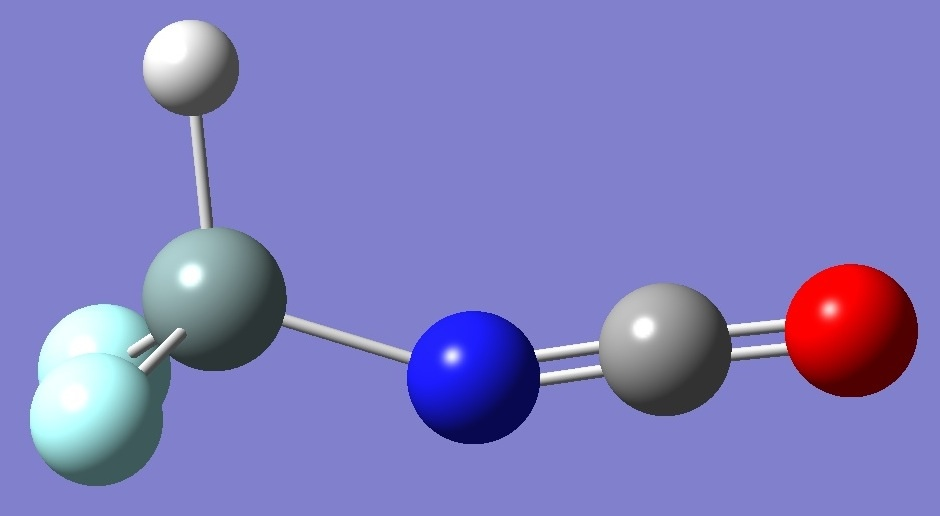HF2SiN=C=O

Nitrogen

Nuclear Quadrupole Coupling Constants

in Difluoroisocyanato Silane

Calculation of the nitrogen nqcc's in difluoroisocyanato silane was made on molecular structures given by HF/6-31G(3d,3p), HF/6-311G(3d,3p), and CCSD(T)/6-311++G(3df,3pd) optimization, the latter derived by Guirgis et al. .  These are compared with the experimental nqcc's  in Table 1.  Structure parameters in Z-matrix format are given in Table 2, rotational constants in Table 3.

In Table 1, RMS is the root mean square difference between calculated and experimental diagonal nqcc's (percentage of average experimental nqcc).  RSD is the residual stand deviation of calibration of the B3PW91/6-311+G(df,pd) model for calculation of the efg's/nqcc's.

 Table 1. 14N nqcc's in HF2SiN=C=O (MHz).  Calculation was made on the (1) HF/6-31G(3d,3p), (2) HF/6-311G(3d,3p), and (3) CCSD(T)/6-311++G(3df,3pd) optimized structures. Calc (1) Calc (2) Calc (3) Expt.  Xaa 1.906 1.878 2.003 1.8833(27) Xbb - 0.980 - 0.982 - 1.053 - 0.9524(32) * Xcc - 0.926 - 0.896 - 0.950 - 0.9309(32) * |Xac| 0.263 0.152 0.048 RMS 0.021 (1.7 %) 0.027 (2.1 %) 0.091 (7.3 %) RSD 0.030 (1.3 %) 0.030 (1.3 %) 0.030 (1.3 %) Xxx - 0.950 - 0.904 - 0.950 Xyy - 0.980 - 0.982 - 1.053 Xzz 1.930 1.886 2.004 ETA 0.0155 0.0414 0.0514 Øz,a 5.27 3.13 0.94 Øa,N=C 0.23 4.86 6.28 Øz,N=C 5.04 7.99 7.22

* Derived here from experimental Xaa and Xbb - Xcc = -0.0214(58) MHz.

Table 2.  HF2SiN=C=O structure parameters (Å and degrees):  HF/6-31G(3d.3p), HF/6-311G(3d.3p), and CCSD(T)/6-311++G(3df,3pd).H
Si,1,R1
N,2,R2,1,A1
C,3,R3,2,A2,1,D1
O,4,R4,3,A3,1,D2
F,2,R5,3,A4,1,D3
F,2,R6,3,A5,1,D4

HF/6-31G(3d.3p)
HF/6-311G(3d.3p)  CCSD(T)

R1=1.44842021
R2=1.67292676
R3=1.18313768
R4=1.14113117
R5=1.56377129
R6=1.56377129
A1=112.56785585
A2=166.13574368
A3=178.90494955
A4=108.63362561
A5=108.63362561
D1=0.
D2=180.
D3=121.88389266
D4=-121.88389266
R1=1.44986651
R2=1.67706189
R3=1.18267873
R4=1.13673596
R5=1.56332
R6=1.56332
A1=112.93244485
A2=157.018334
A3=178.17530274
A4=108.59591867
A5=108.59591867
D1=0.
D2=180.
D3=121.78671115
D4=-121.78671115
R1=1.456
R2=1.687
R3=1.206
R4=1.165
R5=1.579
R6=1.579
A1=112.6
A2=154.6
A3=-177.4
A4=108.6
A5=108.6
D1=0.
D2=0.
D3=121.6
D4=-121.6

 Table 3.  HF2SiN=C=O.  Rotational Constants (MHz). HF/6-31G(3d,3p) HF/6-311G(3d,3p) Expt  A 7210.2 7266.9 7111.28104(179) B 1544.1 1553.7 1565.77581(49) C 1338.7 1343.1 1347.52275(77)

 G.A.Guirgis, Z.Wang, J.Lirjoni, M.H.Palmer, D.Obeenchain, R.A.Peebles, S.A.Peebles, J.Mol.Struct. 983,5(2010).

HNCO
CH3CH2NCO CH3SiF2NCO CH3-SiHF-NCO

Table of Contents

Molecules/Nitrogen

HF2SiNCO.html

Last Modified 10 Nov 2016